# Points to Curve

2019

If you have a list of points that should be connected and drawn in a curvy way, there are already several mathematical and algorithmical solutions to that. I tried to create my own solution to that problem, because each of the existing has its own pros and cons.

In the image below you see how a sequence of points are connected as a Catmull-Rom spline (green) and with my solution (dark blue). I'll call this O-Spline further on.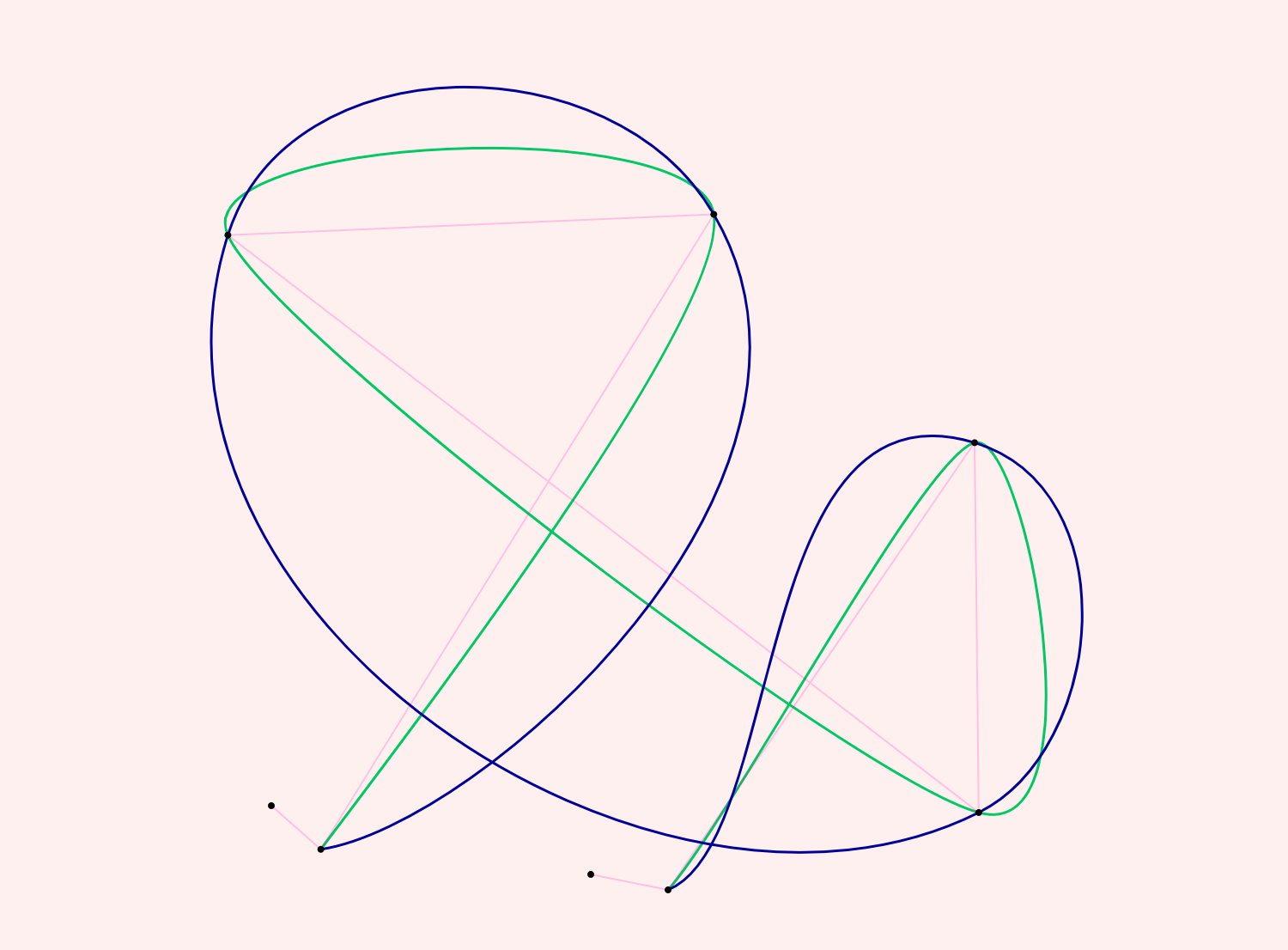You see that the O-Spline takes much longer ways. This is because I wanted it to be as circular as possible in any case. Look at the row of images below. If the points build a regular polygon the Catmull-Rom algorithm sticks closer to that polygon (which might be good in some cases). I wanted the O-Spline to resemble a circle as much as possible.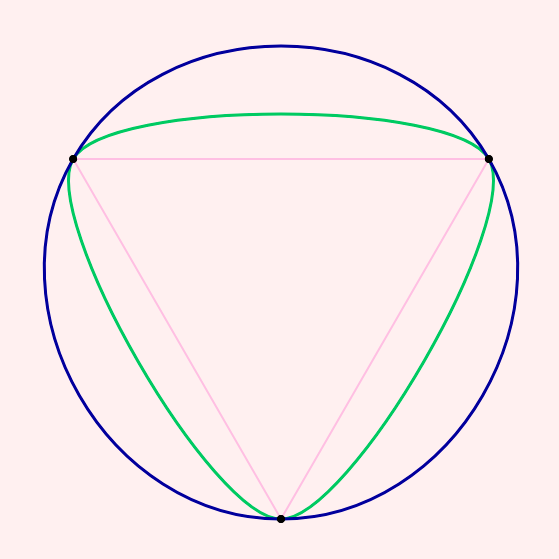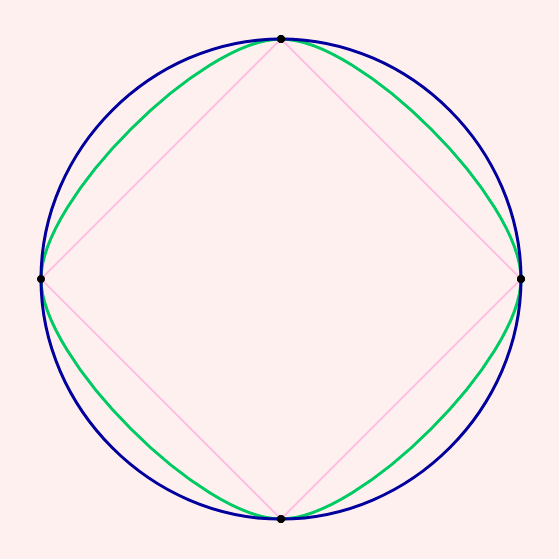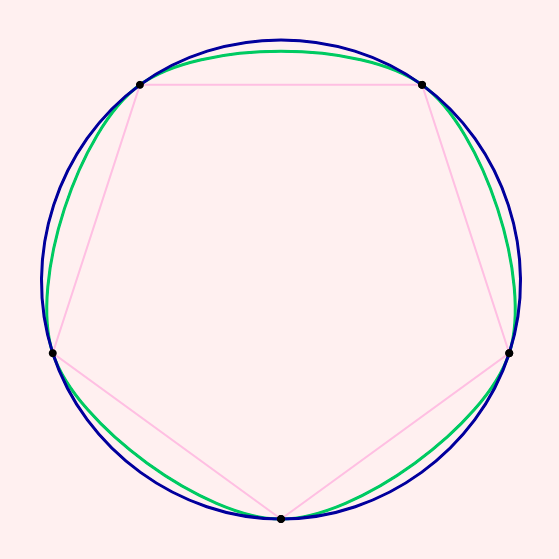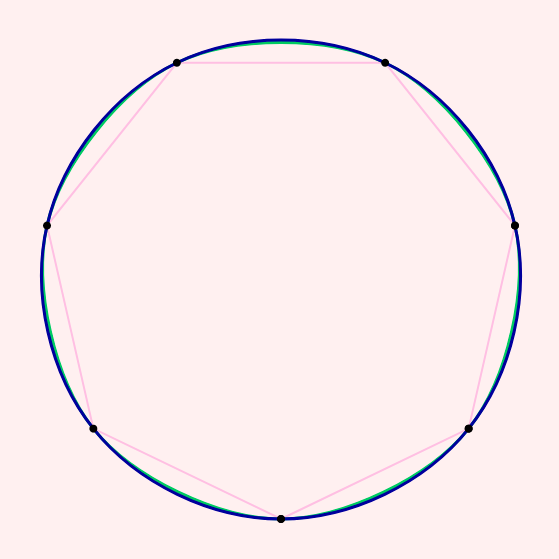Another problem with Catmull-Rom is, that it sometimes generates cusps and intersections within one curve segment and that it could get quite spikey (see image below). The O-Spline is avoiding that.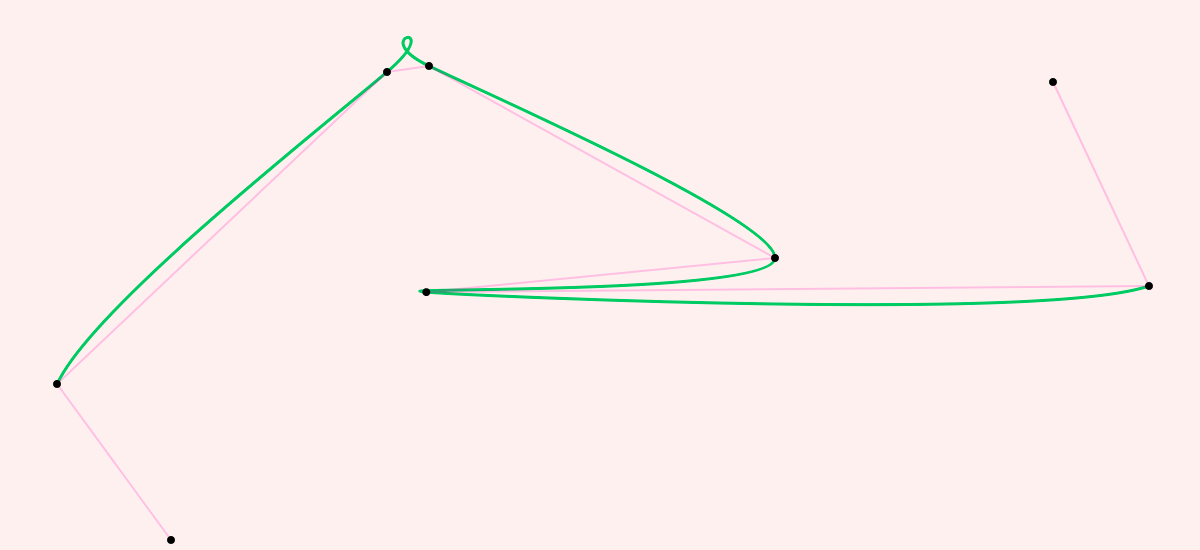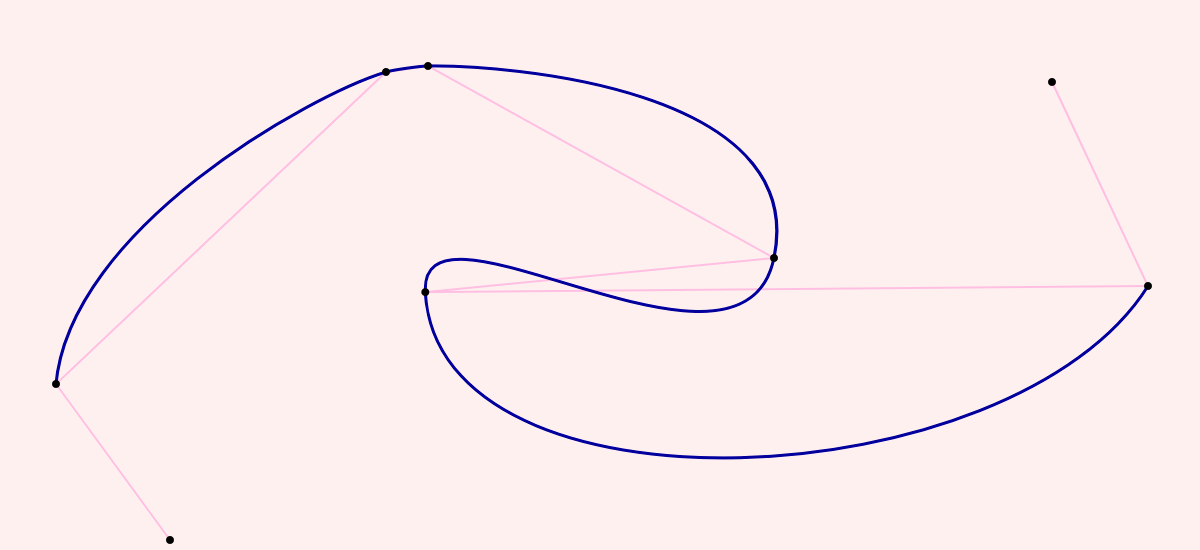One more thing that I didn't like is how Catmull-Rom sometimes looks on more or less straight paths (see below).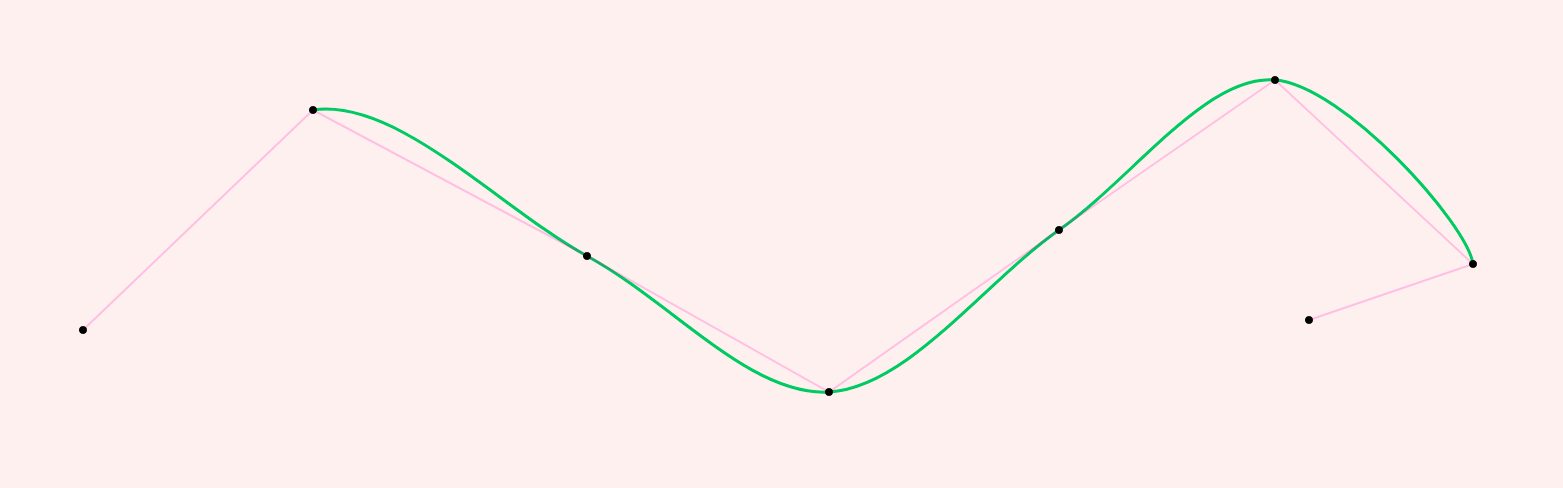The O-Spline turns the tangent on such points a little bit to the right or left to produce a more natural flow: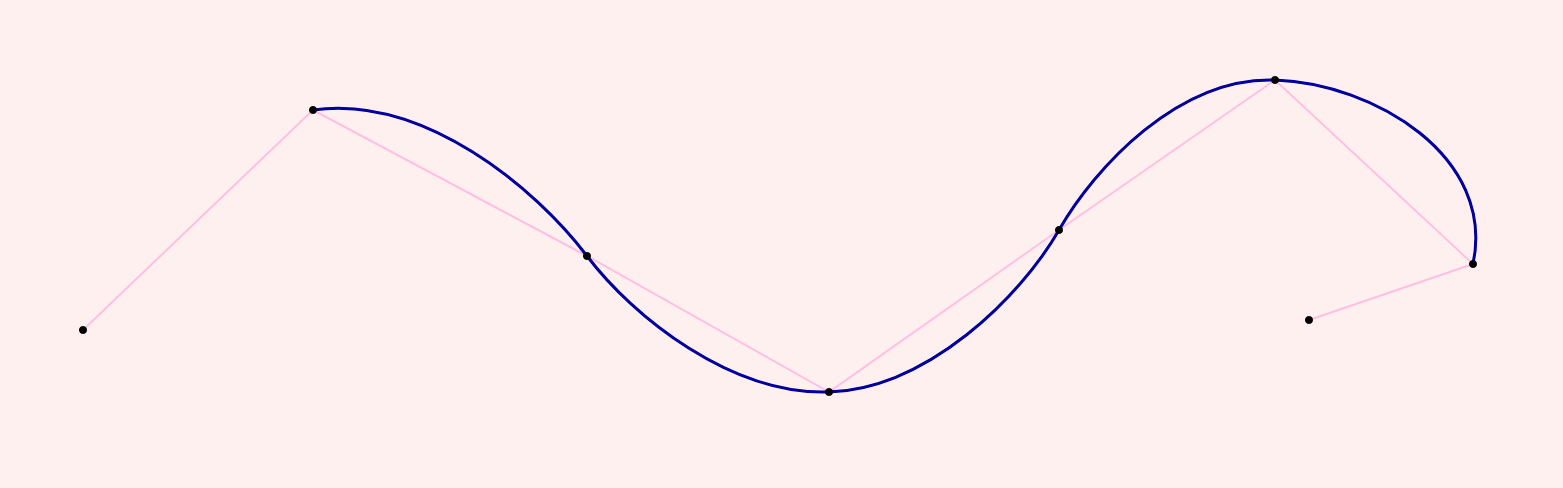## Interactive Demo

In the demo below you can move the points and toggle the display of the paths.

## Some technical remarks

I published the code to produce such an O-Spline as a Gist. It's a function written in plain javascript.

The math for calculating the spline has gotten a bit complicated while refining the algorithm. Maybe it could be simplified.

However, my algorithm is not optimized for performance. If you need to calculate many path segments in very short time, algorithms like Catmull-Rom are a lot faster to calculate.